Hello, readers welcome to new post. In this post we will have a detailed look at Introduction to Linear Dc Machine. Dc machine is a device that operates and generates dc current. The dc generator is such dc machine that generates dc power and dc motor is dc machine that used dc power to work. The common types of dc machines are dc shunt generator, dc series motor and generator, dc compound motor, and generator.

In this post, we discuss the linear dc machines that will help us to understand of practical dc machines. So let get started with LINEAR DC MACHINE.

Introduction to Linear DC Machine

• The linear dc machines is the basic category of dc machine it working operation and other parameters are related to the practical dc machines like motor and generators
• The linear dc machine can be seen in below figure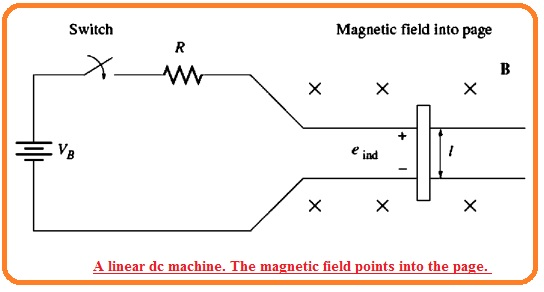• It comprises of battery with a resistor linked with each in the use of a switch that makes frictionless rails.
• The expression of the force applying on the wire in the existence of magnetic field is mention here.

F i(I X B)

Here

• F denotes the force applying on wire
i is the magnitude of current flowing in wire
I is the length of wire,
B is the magnetic flux density
• The expression of the voltage produced in the moving conductive wire is in the field is mentioned here.

eind= (vXB) .l

• eind denotes voltage  in the wire
v denotes the  velocity of the wire
B indicates magnetic flux density
I is the length of the conductor
• If we apply KVL to the circuit shown in the above figure then we have.

VB – iR – eind = 0

VB=eind+IR=0

• Newton law about the bar will be.

Fnet=ma

### Starting Condition of Linear DC Machine

• The diagram shown below indicates the linear dc machine starting requirements.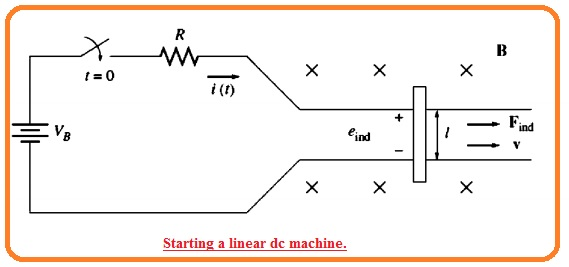• For starting the machine just push the switch. In result, current flows in the conductor that can be friends through the KVL.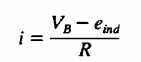• As the conductor bar is in the static state a start eind or induced voltage value is zero. In a result above equation become i=VB/R
• The current passing in the conductor through the tracks.
• According to equation F i(I X B) the current passing in the conductive wire in the existence of magnetic field generates force at the conductor wire.
• Since the structure of the machine, the equation for force is.

Find = ilB at right side

• So the conductor will move in right side according to newton’s law. Though the velocity of the conductor start to increase there is voltage that exists about the conductor.
• Now voltage equation is

eind=vbl in up direction

• The voltage decrease the current passing in the conductor applying the KVL we have.• so eind rises the current will reduces according to above equation.
• In consequence, the conductor will now in constant static condition here total force at the bar is 0
• This exist when eind is rise to the value of VB.
• For this condition, the bar will ahs speed of movement

VB = eind = vssBI

Vss=VB/Bl

• The conductive bar will last to continue this speed when zero load link to it till exterior force is applied to it.
• At start of motor the velocity v produced the voltage eind current and force shown in below figure.• The conclusion of linear dc machine starting conditions is mentioned here.
• If the switch is pushed then generates current movement in the i=VB/R,
• The current generation force at the conductor is mentioned here F=ilB.
• The conductor move to the right side generating an induced voltage.
• The voltage generation decreases the current movement i=(VB-eind)/R
• The force produced is now reduced this condition is also followed by the practical dc machine.

### How Linear DC Machine work as a Motor

• Suppose that at the start there is no load linked to the linear machine according to the requirements discuss above.
• If the load is connected to it then when outcomes will be.
• TO observe this see the below diagram,• In this condition, Fload is given to the conductor that is reverse to the motion direction.
• AS the conductor at the start is in the steady condition the force applied Fload will outcomes in total force at the bar in the direction contrary to the movement direction.
• (F net = Flaod – Find)
• The factor of this force cause to decrement in the speed of the conductor  Like the start it speeds decrease the induced voltage at the conductor decreases.
• with the voltage decrement current also decreases.• So the induced force also increases The net outcome of this happening is the induced force increase till it becomes similar to the force of load and conductor then moves in steady condition now it has less speed.
• If laod is connected to the bar the velocity induced voltage current and induced force can now seen here.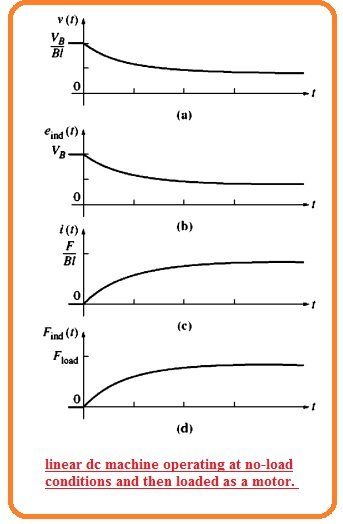• There is induced force in the direction of movement of conductor and power is then transformed from electric to mechanical for retaining the conductor in movement condition.
• The power now transformed to.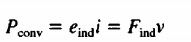The conclusion of the liner machine as dc motor is mentioned here.That is a detailed post about linear dc machines if you have any further query ask in the comments. Thanks for reading have a nice day.